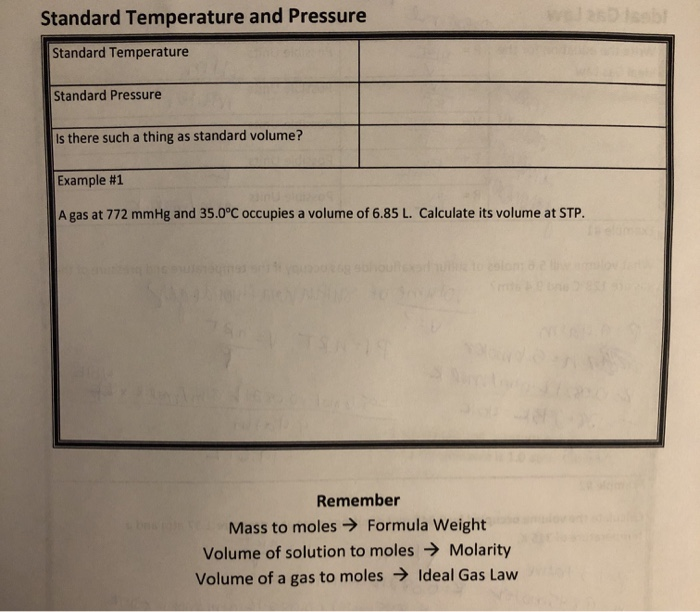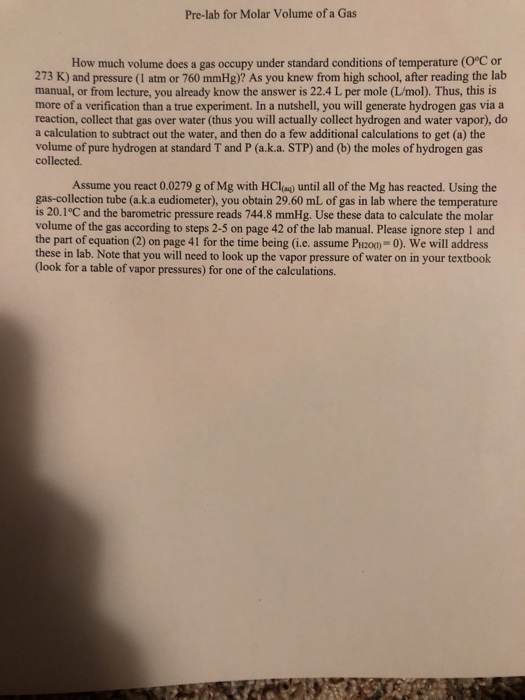# Using the Ideal Gas Law, what volume in L would 1 mole of an ideal gas occupy at standard temperature and pressure? Reme... related homework questions

• #### It’s review question, I need this as soon as possible. Thank you 3) For thè diferential equation: (a) The point zo =-1 is an ordinary point. Compute the recursion formula for the coefficients of...It’s review question, I need this as soon as possible. Thank you 3) For thè diferential equation: (a) The point zo =-1 is an ordinary point. Compute the recursion formula for the coefficients of the power series solution centered at zo- -1 and use it to compute the first three nonzero terms of the power series when -1)-s and v(-1)-0....

• #### Using the Ideal Gas Law, what volume in L would 1 mole of an ideal gas occupy at standard temperature and pressure? Reme...

Using the Ideal Gas Law, what volume in L would 1 mole of an ideal gas occupy at standard temperature and pressure? Remember: STP is 273.15 K and 101.325 kPa.

• #### Dont copié formé thé book oh ya dont copié formé thé book cause you Oiil inde up being triste soi remembré not toi copié frome thé book oh ya

Dont copié formé thé book oh ya dont copié formé thé book cause you Oiil inde up being triste soi remembré not toi copié frome thé book oh ya!translation in english please!

• #### DSuppose \$39oo is deposited in a savings account that increases exponentially.Detamine thě APv if the acount...DSuppose \$39oo is deposited in a savings account that increases exponentially.Detamine thě APv if the acount increases to \$t020 in 4 years. Ass ume tne interest Vale remains Constant and no additional deposits or Withdrawals are made. (a.) Let pbe the APY. Note tnat if tme inital balaqe is yo, ne year later tne balane is %more. P- 3 (Tpe...

• #### Standard Temperature and Pressure Standard Temperature Standard Pressure Is there such a thing as standard volume?...Standard Temperature and Pressure Standard Temperature Standard Pressure Is there such a thing as standard volume? Example #1 A gas at 772 mmHg and 35.0°C occupies a volume of 6.85 L. Calculate its volume at STP. Remember Mass to moles → Formula Weight Volume of solution to moles → Molarity Volume of a gas to moles → Ideal Gas Law

• #### Pre-lab for Molar Volume of a Gas How much volume does a gas occupy under standard conditions of temperature (O°C o...Pre-lab for Molar Volume of a Gas How much volume does a gas occupy under standard conditions of temperature (O°C or 273 K) and pressure (1 atm or 760 mmHg)? As you knew from high school, after reading the lab manual, or from lecture, you already know the answer is 22.4 L per mole (L/mol). Thus, this is more of...

• #### The ideal gas law relates the temperature, pressure and volume of an ideal gas. Suppose the...

The ideal gas law relates the temperature, pressure and volume of an ideal gas. Suppose the gas inside a particular balloon has an absolute pressure of 3.15×105 Pa and occupies a volume of 5.33×10-3 m3 at a temperature of 16.7°C. How many moles of gas are inside the balloon? (do not enter units) How many molecules of gas are inside...

Free Homework App